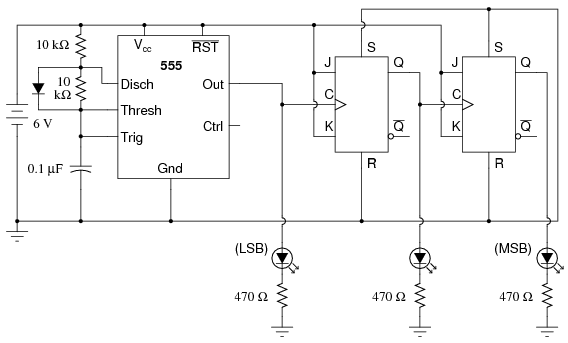# Basic Battery Monitor Circuit, need quick check!

The picture is attached. Sorry if the picture is blurry, the small part(if someone can't see it properly) is a 10k-10k voltage divider. And the middle pin on the Arduino, says analog.
I just need to make sure that everything is connected properly before I wire it. I don't want to break anything and waste time.

Manan

Um maybe your drawing confused me...
What voltages are we dealing with, max voltage being?

The max voltage of the battery will be 8.4V. The divider splits that into 4.2. That voltage will be connected to the analog pin.

EDIT: this is because the analog pin can only read between (0-5) V.

Hi,
I used 2 x 3.7v Lith-ion 14500/AA batteries on my robot/buggy. I too used 2 x 10K resistors across the supply with the centre to an Analogue pin, and then used used this code:

``````{
unit=0.00488;                  //4.88mV per analoge step
bv=(unit*val)*2;               // As we are only reading half the voltage x2!
lcd.clear();
lcd.setCursor(1,0);
lcd.print("Battery Status");
val=bv;
if(val>4.80)
{
lcd.setCursor(1,1);
lcd.print(val);
lcd.print(" V+ -Full");
delay(2000);
factor=1;
}
if(val<=4.80)
{
lcd.setCursor(1,1);
lcd.print(val);
lcd.print(" V -Good");
delay(1000);
factor=1.2;
}
if (val <4.50 and val>4.0)
{
lcd.setCursor(1,1);
lcd.print(val);
lcd.print(" V -OK ?");
delay(1000);
factor=1.4;
}
if (val <4.0 and val>3.70)
{
lcd.setCursor(1,1);
lcd.print(val);
lcd.print(" V -Low");
delay(1000);
factor=1.6;
}
if (val <3.70)
{
lcd.setCursor(0,1);
lcd.print(val);
lcd.print(" V -Recharge!");
delay(3000);

}
``````

I don't see any problems, hope it helps.
Regards

Mel.

Great thanks!
Im going to use a 10LED bar graph.
EDIT: I am not the greatest coder. I am guessing the map function could have also been used. The values I used were derived mathematically on paper
So if anyone wants, here is my code for it:

``````int analogpin = A0;
int analogvalue;
int misc=0;

void setup(){
for(int i= 33; i<53; i+=2)
{
pinMode(i, OUTPUT);
}
Serial.begin(9600);
}

void loop(){
if(analogvalue>=839)
{
misc = 10;
}
else if(analogvalue >=819 && analogvalue <839)
{
misc = 9;
}
else if(analogvalue>=799 && analogvalue <819)
{
misc = 8;
}
else if(analogvalue>=779 && analogvalue < 799)
{
misc = 7;
}
else if(analogvalue>=759 && analogvalue <779)
{
misc = 6;
}
else if(analogvalue>=739 && analogvalue <759)
{
misc = 5;
}
else if(analogvalue>=719 && analogvalue <739)
{
misc = 4;
}
else if(analogvalue>=699 && analogvalue <719)
{
misc = 3;
}
else if(analogvalue>=679 && analogvalue <699)
{
misc = 2;
}
else
misc = 1;

misc*=2;
misc+=33;
for(int i = 33; i<misc; i+=2)
{
digitalWrite(i, HIGH);
}

}
``````

Not so good:Ugh: